Chapter 8: Gas Absorption¶

Ex8.1: Page 278¶

In :
# Illustration 8.1
# Page: 278

print'Illustration 8.1 -  Page: 278\n\n'

# solution

#****Data****#
P_star = 2*10**(5);# [N/square m]
X_methane = 0.6;
X_ethane = 0.2;
X_propane = 0.08;
X_nbutane = 0.06;
X_npentane = 0.06;
#******#

MoleFraction = [0.6, 0.2 ,0.08, 0.06 ,0.06]
Heading = ["Component", "Equilibrium Partial Pressure", "Vapour Pressue    " ,"Mole Fraction"];
Component = ["Methane", "Ethane   " ,"Propane" ,"n-Butane", "n-Pentane"];
VapPressure = [0 ,42.05, 8.96, 2.36 ,0.66];# [N/square m]
Sum = 0;

for i in range(0,5):
print "\n ",Component[i]," \t \t \t \t \t",("{:.2e}".format(MoleFraction[i]*P_star)),"\t \t \t \t \t \t  \t \t ",("{:.2e}".format(VapPressure[i]*10**(5))),
if  VapPressure[i]==0:
Sum = Sum+0;
else:

print "\t \t \t \t \t \t \t \t \t \t",("{:.2e}".format((MoleFraction[i]*P_star)/(VapPressure[i]*10**(5)))),"\t",
Sum = Sum+(MoleFraction[i]*P_star)/(VapPressure[i]*10**(5))

print"\n Mole Fraction Of solvent Oil is ",round(1-Sum,3)
Illustration 8.1 -  Page: 278

Component 	 	 	 	Equilibrium Partial Pressure 	 	 	 	Vapour Pressue     	 	 	 	Mole Fraction

Methane  	 	 	 	 	1.20e+05 	 	 	 	 	 	  	 	  0.00e+00
Ethane     	 	 	 	 	4.00e+04 	 	 	 	 	 	  	 	  4.20e+06 	 	 	 	 	 	 	 	 	 	9.51e-03
Propane  	 	 	 	 	1.60e+04 	 	 	 	 	 	  	 	  8.96e+05 	 	 	 	 	 	 	 	 	 	1.79e-02
n-Butane  	 	 	 	 	1.20e+04 	 	 	 	 	 	  	 	  2.36e+05 	 	 	 	 	 	 	 	 	 	5.08e-02
n-Pentane  	 	 	 	 	1.20e+04 	 	 	 	 	 	  	 	  6.60e+04 	 	 	 	 	 	 	 	 	 	1.82e-01
Mole Fraction Of solvent Oil is  0.74

Ex8.2: Page 286¶

In :
# Illustration 8.2
# Page: 286

print'Illustration 8.2 - Page: 286\n\n'

# solution
from scipy.optimize import fsolve
import numpy
import matplotlib.pyplot as plt
%matplotlib inline
#****Data****#
# Absorber:
G = 0.250;# [cubic m/s]
Temp1 = 273+26.0;# [K]
Pt = 1.07*10**(5);# [N/square m]
y1 = 0.02;
x2 = 0.005;
#******#

G1 = G*(273.0/Temp1)*(Pt/(1.0133*10**(5)))*(1/22.41);# [kmol/s]
Y1 = y1/(1-y1);# [kmol benzene/kmol dry gas]
Gs = G1*(1.0-y1);# [kmol dry gas/s]
# For 95% removal of benzene:
Y2 = Y1*0.05;
X2 = x2/(1.0-x2);# [kmol benzene/kmol oil]
# Vapour pressure of benzene:

P_star = 13330.0;# [N/square m]
X_star = numpy.zeros(20);
Y_star = numpy.zeros(20);
j = -1;
for i in range(1,21,1):
j = j+1;
x = i/100.0;
X_star[j] = i/100.0;
def  f27(y):
return (y/(1+y))-(P_star/Pt)*(x/(1+x))
Y_star[j] = fsolve(f27,0.0);

# For min flow rate:
X1 = 0.176;# [kmolbenzene/kmol oil]
DataMinFlow = numpy.array([[X2, Y2],[X1, Y1]]);

plt.plot(X_star,Y_star,label="Equlibrium Line")
plt.plot(DataMinFlow[:,0],DataMinFlow[:,1],label="Min Flow Rate Line");
minLs = (Gs*(Y1-Y2)/(X1-X2));# [kmol/s]
# For 1.5 times the minimum:
Ls = 1.5*minLs;# [kmol/s]
X1_prime = (Gs*1.0*(Y1-Y2)/Ls)+X2;# [kmol benzene/kmol oil]
DataOperLine = numpy.array([[X2 ,Y2],[X1_prime ,Y1]]);
plt.plot(DataOperLine[:,0],DataOperLine[:,1],label="Operating Line")
plt.grid('on');
xlabel("moles of benzene / mole wash oil");
ylabel("moles benzene / mole dry gas");
legend(loc='lower right');
plt.title("Absorption")
plt.show()
print"The Oil circulation rate is ",("{:.2e}".format(Ls))," kmol/s\n"

# Stripping
Temp2 = 122+273;# [K]
# Vapour pressure at 122 OC
P_star = 319.9;# [kN/square m]
Pt = 101.33;# [kN/square m]
X_star = numpy.zeros(7);
Y_star = numpy.zeros(7);
j = -1;
for i in range(0,7,1):
j = j+1;
x = i/10.0;
X_star[j] = i/10.0;
def f28(y):
return (y/(1.0+y))-(P_star/Pt)*(x/(1.0+x))
Y_star[j] = fsolve(f28,0.0);

X1 = X2;# [kmol benzene/kmol oil]
X2 = X1_prime;# [kmol benzene/kmol oil]
Y1 = 0.0;# [kmol benzene/kmol steam]
# For min. steam rate:
Y2 = 0.45;
DataMinFlow =numpy.array([[X2 ,Y2],[X1 ,Y1]]);
minGs = Ls*(X2-X1)/(Y2-Y1);# [kmol steam/s]
slopeOperat = 1.5*(Y2-Y1)/(X2-X1);
def f29(x):
return slopeOperat*(x-X1)+Y1
x =numpy.arange(0,0.14,0.01)

plt.plot(Y_star,X_star,label="Equlibrium Line")
plt.plot(DataMinFlow[:,0],DataMinFlow[:,1],label="Min Flow Rate Line")
plt.plot(x,f29(x),label="Operating Line");
plt.grid('on');
xlabel("moles of benzene / mole wash oil");
ylabel("moles benzene / mole dry gas");
plt.legend(loc='lower left');
plt.title("Stripping");
plt.show()
print"The Steam circulation rate is ",("{:.2e}".format(1.5*minGs))," kmol/s\n"
#the answers are slightly different in textbook due to approximation while here answers are precise
Illustration 8.2 - Page: 286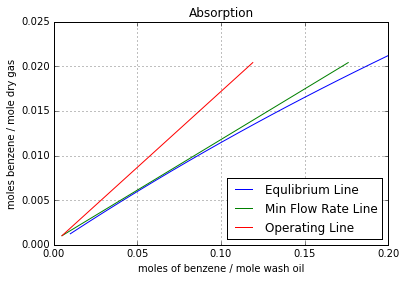The Oil circulation rate is  1.79e-03  kmol/s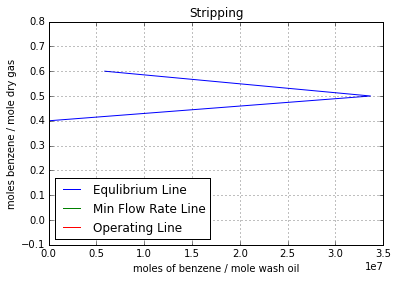The Steam circulation rate is  6.81e-04  kmol/s

Ex8.3: Page 292¶

In :
# Illustration 8.3
# Page: 292

print'Illustration 8.3 - Page: 292\n\n'

# solution

import math
# Since tower is a tray device:
# Following changes in notation is made:
# L1 to LNp
# L2 to L0
# X1 to XNp
# X2 to X0
# G1 to GNpPlus1
# G2 to G1
# Y1 to YNpPlus1
# Y2 to Y1
# x1 to xNp
# x2 to x0
# y1 to yNpPlus1
# y2 to y1
# From Illustration 8.2:
yNpPlus1 = 0.02;
Y1 = 0.00102;
y1 = Y1/(1+Y1);
GNpPlus1 = 0.01075;# [kmol/s]
x0 = 0.005;
m = 0.125;# [m = y_star/x]
Ls = 1.787*10**(-3);# [kmol/s]
Gs = 0.01051;# [kmol/s]
XNp = 0.1190;
LNp = Ls*(1+XNp);# [kmol/s]
ANp = LNp/(m*GNpPlus1);
X0 = x0/(1-x0);
L0 = Ls*(1+X0);# [kmol/s]
G1 = Gs*(1+Y1);# [kmol/s]
A1 = L0/(m*G1);
A = (ANp*A1)**0.5;
# From Eqn. 5.55:
Np = (math.log((yNpPlus1-(m*x0))/(y1-(m*x0))*(1-(1/A))+(1/A)))/math.log(A);
print"Absorber\n"
print"From Analytical Method, no. of theoretical trays required is  \n",round(Np,4)
# From Fig. 8.13 (Pg292):
Np = 7.6;
print"From Graphical Method, no. of theoretical trays required is \n",Np

# Stripper
SNp = 1/ANp;
S1 = 1/A1;
# Due to relative nonconstancy of the stripping factor,graphical method should be used.
print"Stripper\n"
# From Fig. 8.11 (Pg 289):
Np = 6.7;
print"From Graphical Method, no. of theoretical trays required is \n",Np
# From Fig. 5.16 (Pg 129):
Np = 6.0;
print"From Fig. 5.16, no. of theoretical trays required is \n",Np
Illustration 8.3 - Page: 292

Absorber

From Analytical Method, no. of theoretical trays required is
7.7085
From Graphical Method, no. of theoretical trays required is
7.6
Stripper

From Graphical Method, no. of theoretical trays required is
6.7
From Fig. 5.16, no. of theoretical trays required is
6.0

Ex8.4: Page 295¶

In :
# Illustration 8.4
# Page: 295

print'Illustration 8.4 - Page: 295\n\n'

# solution
import math
import numpy
from scipy.optimize import fsolve
import matplotlib.pyplot as plt
%matplotlib inline
#****Data****#
# a = CH4 b = C5H12
Tempg = 27.0;# [OC]
Tempo = 0.0;# [base temp,OC]
Templ = 35.0;# [OC]
xa = 0.75;# [mole fraction of CH4 in gas]
xb = 0.25;# [mole fraction of C5H12 in gas]
M_Paraffin = 200.0;# [kg/kmol]
hb = 1.884;# [kJ/kg K]
#********#

Ha = 35.59;# [kJ/kmol K]
Hbv = 119.75;# [kJ/kmol K]
Hbl = 117.53;# [kJ/kmol K]
Lb = 27820;# [kJ/kmol]
# M = [Temp (OC) m]
M = numpy.array([[20 ,0.575],[25 ,0.69],[30 ,0.81],[35, 0.95],[40, 1.10],[43, 1.25]]);
# Basis: Unit time
GNpPlus1 = 1.0;# [kmol]
yNpPlus1 = 0.25;# [kmol]
HgNpPlus1 = ((1-yNpPlus1)*Ha*(Tempg-Tempo))+(yNpPlus1*(Hbv*(Tempg-Tempo)+Lb));# [kJ/kmol]
L0 = 2.0;# [kmol]
x0 = 0.0;# [kmol]
HL0 = ((1-x0)*hb*M_Paraffin*(Templ-Tempo))+(x0*hb*(Templ-Tempo));# [kJ/kmol]
C5H12_absorbed = 0.98*xb;# [kmol]
C5H12_remained = xb-C5H12_absorbed;
G1 = xa+C5H12_remained;# [kmol]
y1 = C5H12_remained/G1;# [kmol]
LNp = L0+C5H12_absorbed;# [kmol]
xNp = C5H12_absorbed/LNp;# [kmol]
# Assume:
Temp1 = 35.6;# [OC]
Hg1 = ((1-y1)*Ha*(Temp1-Tempo))+(y1*(Hbv*(Temp1-Tempo)+Lb));# [kJ/kmol]

# Eqn. 8.11:
Qt = 0;
def f30(HlNp):
return ((L0*HL0)+(GNpPlus1*HgNpPlus1))-((LNp*HlNp)+(G1*Hg1)+Qt)
HlNp = fsolve(f30,2);

def f31(TempNp):
return HlNp-(((1-x0)*hb*M_Paraffin*(TempNp-Tempo))+(x0*hb*(TempNp-Tempo)))
TempNp = fsolve(f31,35.6);
# At Temp = TempNp:
mNp = 1.21;
yNp = mNp*xNp;# [kmol]
GNp = G1/(1-yNp);# [kmol]
HgNp = ((1-yNp)*Ha*(TempNp-Tempo))+(yNp*(Hbv*(TempNp-Tempo)+Lb));# [kJ/kmol]
# Eqn. 8.13 with n = Np-1
def f32(LNpMinus1):
return LNpMinus1+GNpPlus1-(LNp+GNp)
LNpMinus1 = fsolve(f32,2);# [kmol]

# Eqn. 8.14 with n = Np-1
def f33(xNpMinus1):
return ((LNpMinus1*xNpMinus1)+(GNpPlus1*yNpPlus1))-((LNp*xNp)+(GNp*yNp))
xNpMinus1 = fsolve(f33,0);# [kmol]

# Eqn. 8.15 with n = Np-1
def  f34(HlNpMinus1):
return ((LNpMinus1*HlNpMinus1)+(GNpPlus1*HgNpPlus1))-((LNp*HlNp)+(GNp*HgNp))
HlNpMinus1 = fsolve(f34,0);# [kJ/kmol]
def f35(TempNpMinus1):
return HlNpMinus1-(((1-xNpMinus1)*hb*M_Paraffin*(TempNpMinus1-Tempo))+(xNpMinus1*hb*(TempNpMinus1-Tempo)))
TempNpMinus1 = fsolve(f35,42);# [OC]

# The computation are continued upward through the tower in this manner until the gas composition falls atleast to 0.00662.
# Results = [Tray No.(n) Tn(OC) xn yn]
Results = numpy.array([[4.0 ,42.3 ,0.1091 ,0.1320],[3 ,39.0, 0.0521 ,0.0568],[2 ,36.8 ,0.0184 ,0.01875],[1 ,35.5, 0.00463 ,0.00450]]);

plt.plot(Results[:,0],Results[:,3]);
plt.grid('on');
xlabel('Tray Number');
ylabel('mole fraction of C5H12 in gas');
plt.show();
plt.plot(Results[:,0],Results[:,1]);
plt.grid('on');
xlabel('Tray Number');
ylabel('Temperature(OC)');
plt.show();

# For the required y1
Np = 3.75;
print"The No. of trays will be ",Np
Illustration 8.4 - Page: 295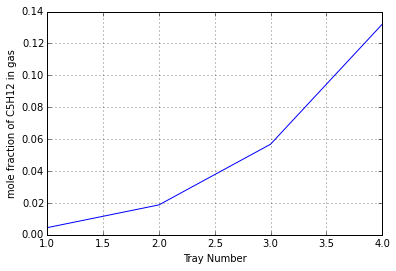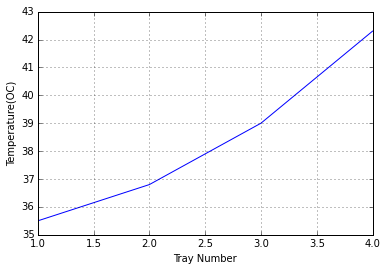The No. of trays will be  3.75

Ex8.5: Page 299¶

In :
# Illustration 8.5
# Page: 299

print'Illustration 8.5 - Page: 299\n\n'

# solution

import math
import numpy
from scipy.optimize import fsolve
#****Data****#
# a = NH3 b = H2 c = N2 w = water
P = 2.0;# [bars]
Temp = 30.0;# [OC]
L = 6.38;# [kg/s]
W = 0.53;# [weir length,m]
pitch = 12.5/1000;# [m]
D = 0.75;# [Tower diameter,m]
hW = 0.060;# [weir height,m]
t = 0.5;# [tray spacing,m]
#*******#

# From Geometry of Tray Arrangement:
At = 0.4418;# [Tower Cross section,square m]
Ad = 0.0403;# [Downspout Cross section,square m]
Ao = 0.0393;# [perforation area,square m]
Z = 0.5307;# [distance between downspouts,square m]
z = (D+W)/2.0;# [average flow width,m]
h1 = 0.04;# [weir crest,m]
# From Eqn. 6.34
Weff = W*(math.sqrt(((D/W)**2)-((((D/W)**2-1)**0.5)+((2*h1/D)*(D/W)))**2));# [m]
q = Weff*(1.839*h1**(3/2));#[cubic m/s]
# This is a recommended rate because it produces the liquid depth on the tray to 10 cm.
Density_L = 996;# [kg/s]
Mw = 18.02;# [kg/kmol]
L1 = 6.38/Mw;# [kmol/s]
Ma = 17.03;# [kg/kmol]
Mb = 28.02;# [kg/kmol]
Mc = 2.02;# [kg/kmol]
MavG = (0.03*Ma)+(0.97*(1/4)*Mb)+(0.97*(3/4)*Mc);# [kg/kmol]
Density_G = (MavG/22.41)*(P/0.986)*(273/(273+Temp));# [kg/cubic m]
G = 0.893;# [kg/s]
sigma = 68*10**(-3);# [N/m]
abcissa = (L/G)*(Density_G/Density_L)**0.5;
# From Table 6.2 (Pg169):
alpha = 0.04893;
beeta = 0.0302;
# From Eqn. 6.30
Cf = ((alpha*math.log10(1.0/abcissa))+beeta)*(sigma/0.02)**0.2;
# From Eqn. 6.29
Vf = Cf*((Density_L-Density_G)/Density_G)**(1.0/2);# [m/s]
# 80% of flooding value:
V = 0.8*Vf;# [m/s]
G = 0.8*G;# [kg/s]
G1 = G/MavG;# [kmol/s]
Vo = V*An/Ao;# [m/s]
l = 0.002;# [m]
Do = 0.00475;# [m]
# From Eqn. 6.37
Co = 1.09*(Do/l)**0.25;
viscosity_G = 1.13*10**(-5);# [kg/m.s]
Reo = Do*Vo*Density_G/viscosity_G;
# At Reynold's No. = Reo
fr = 0.0082;
g = 9.81;# [m/s^2]
# From Eqn. 6.36
def f36(hD):
return (2*hD*g*Density_L/(Vo**2*Density_G))-(Co*(0.40*(1.25-(Ao/An))+(4*l*fr/Do)+(1-(Ao/An))**2))
hD = fsolve(f36,1);
# From Eqn. 6.31;
Aa = (Ao/0.907)*(pitch/Do)**2;# [square m]
Va = V*An/Aa;# [m/s]
# From Eqn. 6.38
hL = 6.10*10**(-3)+(0.725*hW)-(0.238*hW*Va*(Density_G)**0.5)+(1.225*q/z);# [m]
# From Eqn. 6.42
hR = 6*sigma/(Density_L*Do*g);# m
# From Eqn. 6.35
hG = hD+hL+hR;# [m]
Al = 0.025*W;# [square m]
# From Eqn. 6.43
# From Eqn.6.44
h3 = hG+h2;
# since hW+h1+h3 is essentially equal to t/2, flooding will not occur
abcissa = (L/G)*(Density_G/Density_L)**0.5;
V_by_Vf = V/Vf;
# From Fig.6.17, V/Vf = 0.8 & abcissa = 0.239
E = 0.009;

# At the prevailing conditions:
Dg = 2.296*10**(-5);# [square m/s]
viscosity_G = 1.122*10**(-5);# [kg/m.s]
ScG = viscosity_G/(Density_G*Dg)
Dl = 2.421*10**(-9);# [square m/s]

# From Henry's Law:
m = 0.850;
A = L1/(m*G1);

# From Eqn. 6.61:
NtG = (0.776+(4.57*hW)-(0.238*Va*Density_G**0.5)+(104.6*q/Z))/(ScG**0.5);
# From Eqn. 6.64:
thetha_L = hL*z*Z/q;# [s]
# From Eqn. 6.62:
NtL = 40000*(Dl**0.5)*((0.213*Va*Density_G**0.5)+0.15)*thetha_L;
# From Eqn. 6.52:
NtoG = 1/((1/NtG)+(1/(A*NtL)));
# From Eqn. 6.51:
EoG = 1-math.exp(-NtoG);
# From Eqn. 6.63:
DE = ((3.93*10**(-3))+(0.0171*Va)+(3.67*q/Z)+(0.1800*hW))**2;# [square m/s]
# From Eqn. 6.59:
Pe = Z**2/(DE*thetha_L);
# From Eqn. 6.58:
eta = (Pe/2.0)*((1+(4*m*G1*EoG/(L1*Pe)))**0.5-1);
# From Eqn. 6.57:
EMG = EoG*(((1-math.exp(-(eta+Pe)))/((eta+Pe)*(1+(eta+Pe)/eta)))+((exp(eta)-1)/(eta*(1+(eta/(eta+Pe))))));
# From Eqn. 6.60:
EMGE = EMG/((1+(EMG*(E/(1-E)))));
# From Eqn. 8.16:
EO = math.log(1+EMGE*((1.0/A)-1))/math.log(1.0/A);
Np = 14*EO;
yNpPlus1 = 0.03;
x0 = 0;
# From Eqn. 5.54(a):
def f37(y1):
return ((yNpPlus1-y1)/(yNpPlus1-m*x0))-(((A**(Np+1))-A)/((A**(Np+1))-1))
y1 = fsolve(f37,0.03);
print"Mole Fraction Of NH3 in effluent is ",round(y1,4)
Illustration 8.5 - Page: 299

Mole Fraction Of NH3 in effluent is  0.0211

Ex8.6: Page 304¶

In :
# Illustration 8.6
# Page: 304

print'Illustration 8.6 - Page: 304\n\n'

# solution

import matplotlib.pyplot as plt
%matplotlib inline
import numpy
from scipy.optimize import fsolve
import math
#****Data****#
# Gas:
# In:
y_prime1 = 0.02;
Y_prime1 = 0.0204;# [mol/mol dry gas]
# Out:
y_prime2 = 0.00102;
Y_prime2 = 0.00102;# [mol/mol dry gas]
# Non absorbed gas:
MavG = 11;# [kg/kmol]
G = 0.01051;# [kmol/s nonbenzene]
Gm = 0.01075;# [kmol/s]
T = 26;# [OC]
viscosity_G = 10**(-5);# [kg/m.s]
DaG = 1.30*10**(-5);# [square m/s]

# Liquid:
# In:
x_prime2 = 0.005;
X_prime2 = 0.00503;# [mol benzene/mol oil]
# Out:
x_prime1 = 0.1063;
X_prime1 = 0.1190;# [mol benzene/mol oil]
# Benzene free oil:
MavL = 260.0;# [kg/kmol]
viscosity_L = 2*10**(-3);# [kg/kmol]
Density_L = 840;# [kg/cubic cm]
L = 1.787*10**(-3);# [kmol/s]
DaL = 4.77*10**(-10);# [square m/s]
sigma = 0.03;# [N/square m]
m = 0.1250;
#*******#

A = 0.47**2*math.pi/4;# [square m]
# At the bottom:
L_prime1 = ((L*MavL)+(X_prime1*L*78))/A;# [kg/square m.s]
# At the top
L_prime2 = ((L*MavL)+(X_prime2*L*78))/A;# [kg/square m.s]
L_primeav = (L_prime1+L_prime2)/2;# [kg/square m.s]
# At the bottom
G_prime1 = ((G*MavG)+(Y_prime1*G*78))/A;# [kg/square m.s]
# At the top
G_prime2 = ((G*MavG)+(Y_prime2*G*78))/A;# [kg/square m.s]
G_primeav = (G_prime1+G_prime2)/2;# [kg/square m.s]

# From Illustration 6.6:
Fga = 0.0719;# [kmol/cubic cm.s]
Fla = 0.01377;# [kmol/cubic cm.s]
# Operating Line:
X_prime = numpy.array([0.00503 ,0.02 ,0.04 ,0.06 ,0.08 ,0.10 ,0.1190]);
x_prime = numpy.zeros(7);
Y_prime = numpy.zeros(7);
y_prime = numpy.zeros(7);
for i in range(0,7):
x_prime[i] = X_prime[i]/(1+X_prime[i]);
def f38(Y_prime):
return (G*(Y_prime1-Y_prime))-(L*(X_prime1-X_prime[i]))
Y_prime[i] = fsolve(f38,Y_prime1);
y_prime[i] = (Y_prime[i])/(1+Y_prime[i]);

def f39(x):
return m*x
x = numpy.arange(0,0.14,0.01);

# Interface compositions are determined graphically and according to Eqn. 8.21:
yi = [0.000784, 0.00285, 0.00562 ,0.00830 ,0.01090 ,0.01337 ,0.01580];
ylog = zeros(7);
y_by_yDiffyi = zeros(7);
for i in range(0,7):
ylog[i] = math.log10(yi[i]);
y_by_yDiffyi[i] = y_prime[i]/(y_prime[i]-yi[i]);

plt.plot(x_prime,y_prime,label="Operating Line")
plt.plot(x,f39(x),label="Equilibrium Line")
plt.plot(x_prime,yi,label="Interface Composition");
plt.legend(loc='lower right');
plt.grid('on');
xlabel("mole fraction of benzene in liquid");
ylabel("mole fraction of benzene in gas");
plt.show()
plt.plot(ylog,y_by_yDiffyi);
plt.grid();
xlabel("log y");
ylabel("y/(y-yi)");
title("Graphical Integration Curve");
plt.show()
# Area under the curve:
Ac = 6.556;
# Eqn. 8.28:
NtG = (2.303*Ac)+1.152*(math.log10((1-y_prime2)/(1-y_prime1)));
Gav = (Gm+(G/(1-Y_prime2)))/(2*A);# [kmol/square m.s]
HtG = Gav/Fga;# [m]
Z = HtG*NtG;# [m]
print"The depth of packing required is ",round(Z,3)," m"
Illustration 8.6 - Page: 304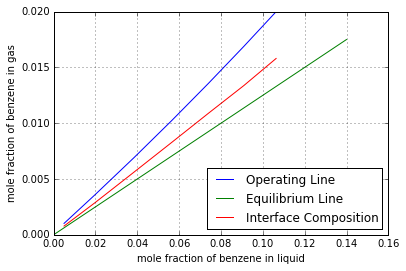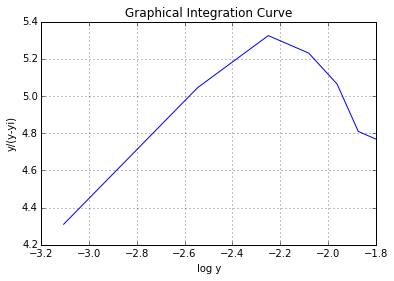The depth of packing required is  12.881  m

Ex8.7: Page 312¶

In :
# Illustration 8.7
# Page: 312

print'Illustration 8.7 - Page: 312\n\n'

# solution

import math
import matplotlib.pyplot as plt
%matplotlib inline
import numpy
from scipy.optimize import fsolve
# Fom Illustration 8.6:
y1 = 0.02;
y2 = 0.00102;
m = 0.125;
x2 = 0.005;
x1 = 0.1063;

# Number of transfer units:
# Method a:
y1_star = m*x1;
y2_star = m*x2;
yDiffy_star1 = y1-y1_star;
yDiffy_star2 = y2-y2_star;
yDiffy_starm = (yDiffy_star1-yDiffy_star2)/math.log(yDiffy_star1/yDiffy_star2);
# From Eqn. 8.48:
NtoG = (y1-y2)/yDiffy_starm;
print"NtoG according to Eqn. 8.48:",round(NtoG,2),"\n"

# Mehod b:
# From Illustration 8.3:
A = 1.424;
NtoG = (math.log((((y1-(m*x2))/(y2-(m*x2)))*(1-(1/A)))+(1/A)))/(1-(1/A));
print"NtoG according to Eqn. 8.50:",round(NtoG,2),"\n"

# Method c:
# Operating Line:
# From Illustration 8.3:
X_prime = [0.00503, 0.02, 0.04 ,0.06 ,0.08 ,0.10 ,0.1190];
x_prime = [0.00502 ,0.01961, 0.0385, 0.0566, 0.0741, 0.0909 ,0.1063]
Y_prime = [0.00102 ,0.00357 ,0.00697 ,0.01036 ,0.01376 ,0.01714 ,0.0204];
y_prime = [0.00102 ,0.00356, 0.00692 ,0.01025 ,0.01356 ,0.01685, 0.0200];
def f2(x):
return  m*x
x = numpy.arange(0,0.14,0.01);

plt.plot(x_prime,y_prime,label="Operating Line")
plt.plot(x,f2(x),label="Equilibrium Line");
plt.legend(loc='upper right');
plt.grid('on');
xlabel("mole fraction of benzene in liquid");
ylabel("mole fraction of benzene in gas");
plt.show()
# From graph:
NtoG = 8.7;
print"NtoG from graph:",round(NtoG,2)," \n",

# Method d:
# from Fig 8.10:
Y_star = [0.000625, 0.00245, 0.00483, 0.00712 ,0.00935 ,0.01149, 0.01347];
ordinate = numpy.zeros(7);
for i in range(0,7):
ordinate[i] = 1/(Y_prime[i]-Y_star[i]);

plt.plot(Y_prime,ordinate);
plt.grid('on');
xlabel("Y");
ylabel("1/(Y-Y*)");
plt.title("Graphical Integration");
plt.show()
# Area under the curve:
Ac = 8.63;
# From Eqn. 8.36:
NtoG = Ac+(1.0/2)*math.log((1+y2)/(1+y1));
print"NtoG from graphical integration:",round(NtoG,2),"\n"

# Height of transfer units:
NtoG = 9.16;
# From Illustration 6.6:
Fga = 0.0719;# [kmol/cubic m.s]
Fla = 0.01377;# [kmol/cubic m.s]
Gav = 0.0609;# [kmol/square m.s]
L = 1.787*10**(-3);# [kmol/s]
X1 = x1/(1-x1);
X2 = x2/(1-x2);
Area = 0.1746;# [square m]
Lav = L*((1+X1)+(1+X2))/(2*Area);
# From Eqn. 8.24:
Htg = Gav/Fga;# [m]
# From Eqn. 8.31:
Htl = Lav/Fla;# [m]
# since Solutions are dilute:
HtoG = Htg+Htl/A;# [m]
print"HtoG: ",round(HtoG,2)," m\n",
Z = HtoG*NtoG;# [m]
print"The depth of packing recquired is ",round(Z,2)," m"
Illustration 8.7 - Page: 312

NtoG according to Eqn. 8.48: 8.51

NtoG according to Eqn. 8.50: 9.16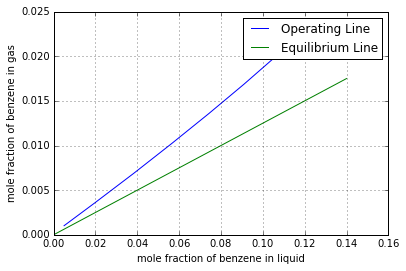NtoG from graph: 8.7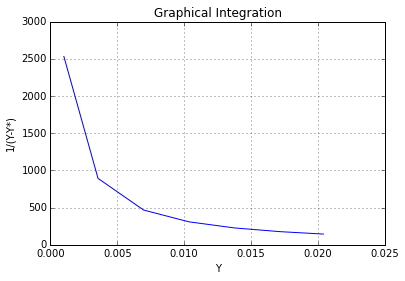NtoG from graphical integration: 8.62

HtoG:  1.4  m
The depth of packing recquired is  12.84  m

Ex8.8: Page 317¶

In :
# Illustration 8.8
# Page: 317

print'Illustration 8.8 - Page: 317\n\n'

# Solution

import math
import matplotlib.pyplot as plt
%matplotlib inline
import numpy
from scipy.optimize import fsolve

#***Data***
# a:NH3 b:air c:H2O
ya = 0.416;# [mole fraction]
yb = 0.584;# [mole fraction]
G1 = 0.0339;# [kmol/square m.s]
L1 = 0.271;# [kmol/square m.s]
TempG1 = 20;# [OC]
#********#

# At 20 OC
Ca = 36390;# [J/kmol]
Cb = 29100;# [J/kmol]
Cc = 33960;# [J/kmol]
lambda_c = 44.24*10**6;# [J/kmol]
# Enthalpy base = NH3 gas, H2O liquid, air at 1 std atm.
Tempo = 20;# [OC]
lambda_Ao = 0;# [J/kmol]
lambda_Co = 44.24*10**6;# [J/kmol]

# Gas in:
Gb = G1*yb;# [kmol air/square m.s]
Ya1 = ya/(1-ya);# [kmol NH3/kmol air]
yc1 = 0;# [mole fraction]
Yc1 = yc1/(1-yc1);# [kmol air/kmol NH]
# By Eqn 8.58:
Hg1 = (Cb*(TempG1-Tempo))+(Ya1*(Ca*(TempG1-Tempo))+lambda_Ao)+(Yc1*(Cc*(TempG1-Tempo)+lambda_Co));# [J/kmol air]

# Liquid in:
xa1 = 0;# [mole fraction]
xc1 = 1;# [mole fraction]
Hl1 = 0;# [J/kmol air]

#Gas out:
Ya2 = Ya1*(1-0.99);# [kmol NH3/kmol air]
# Assume:
TempG2 = 23.9;# [OC]
yc2 = 0.0293;
def  f(Yc2):
return  yc2-(Yc2/(Yc2+Ya2+1))
Yc2 = fsolve(f,0.002);# [kmol H2O/kmol air]
Hg2 = (Cb*(TempG2-Tempo))+(Ya2*(Ca*(TempG2-Tempo))+lambda_Ao)+(Yc2*(Cc*(TempG2-Tempo)+lambda_Co));# [J/kmol air]

# Liquid out:
Lc = L1-(Yc1*Gb);# [kmol/square m.s]
La = Gb*(Ya1-Ya2);# [kmol/square m.s]
L2 = La+Lc;# [kmol/square m.s]
xa = La/L2;
xc = Lc/L2;
# At xa & tempo = 20 OC
delta_Hs = -1709.6*1000;# [J/kmol soln]

# Condition at the bottom of the tower:
# Assume:
TempL = 41.3;# {OC}
# At(TempL+TempG1)/2:
Cl = 75481.0;# [J/kmol]
def f40(Cl):
return  Hl1+Hg1-((Gb*Hg2)+(L2*(Cl*(TempL-Tempo)+delta_Hs)))
Cl = fsolve(f40,7);# [J/kmol.K]

# For the Gas:
MavG = 24.02;# [kg/kmol]
Density_G = 0.999;# [kg/cubic m]
viscosity_G  =  1.517*10**(-5);# [kg/m.s]
kG  =  0.0261;# [W/m.K]
CpG  =  1336;# [J/kg.K]
Dab  =  2.297*10**(-5);# [square m/s]
Dac  =  3.084*10**(-5);# [square m/s]
Dcb  =  2.488*10**(-5);# [square m/s]
PrG  =  CpG*viscosity_G/kG;

# For the liquid:
MavL  =  17.97;# [kg/kmol]
Density_L  =  953.1;# [kg/cubic m]
viscosity_L  =  6.408*10**(-4);# [kg/m.s]
Dal  =  3.317*10**(-9);# [square m/s]
kl = 0.4777;# [W/m.K]
ScL = viscosity_L/(Density_L*Dal);
PrL = 5.72;
sigma = 3*10**(-4);
G_prime = G1*MavG;# [kg/square m.s]
L_prime = L2*MavL;# [kg/square m.s]
# From data of Chapter 6:
Ds = 0.0472;# [m]
a = 57.57;# [square m/cubic m]
shiLt = 0.054;
e = 0.75;
# By Eqn. 6.71:
eLo = e-shiLt;
# By Eqn. 6.72:
kL = (25.1*Dal/Ds)*(Ds*L_prime/viscosity_L)**0.45*ScL**0.5;# [m/s]
c = Density_L/MavL;# [kmol/cubic m]
Fl = kL*c;# [kmol/cubic m]
# The heat mass transfer analogy of Eqn. 6.72:
hL = (25.1*kl/Ds)*(Ds*L_prime/viscosity_L)**0.45*PrL**0.5;# [m/s]
# The heat transfer analogy of Eqn. 6.69:
hG = (1.195*G_prime*CpG/PrG**(2/3))*(Ds*G_prime/(viscosity_G*(1-eLo)))**(-0.36);# [W/square m.K]
# To obtain the mass transfer coeffecients:
Ra = 1.4;
Rc = 1-Ra;
# From Eqn. 8.83:
Dam = (Ra-ya)/(Ra*((yb/Dab)+((ya+yc1)/Dac))-(ya/Dac));# [square m/s]
Dcm = (Rc-yc1)/(Rc*((yb/Dcb)+((ya+yc1)/Dac))-(yc1/Dac));# [square m/s]
ScGa = viscosity_G/(Density_G*Dam);
ScGc = viscosity_G/(Density_G*Dcm);
# By Eqn. 6.69:
FGa = (1.195*G1/ScGa**(2/3))*(Ds*G_prime/(viscosity_G*(1-eLo)))**(-0.36);# [kmol/square m.K]
FGc = (1.195*G1/ScGc**(2/3))*(Ds*G_prime/(viscosity_G*(1-eLo)))**(-0.36);# [kmol/square m.K]
Ra = Ra-0.1;
# From Eqn. 8.80:

for i in range(0,3):
def f41(xai):
return Ra-(Ra-ya)*((Ra-xa)/(Ra-xai))**(Fl/FGa)
xai = numpy.arange(xa,0.10,0.01)
plt.plot(xai,f41(xai))
Ra = Ra+0.1;

plt.grid('on');
xlabel("Mole fraction NH3 in the liquid, xa");
ylabel("Mole fraction NH3 in the gas ya");
title("Operating Line curves");
plt.show()
Rc = Rc-0.1;
# From Eqn. 8.81:

for i  in range(0,3):
def f42(xci):
return Rc-(Rc-yc1)*((Rc-xc)/(Rc-xci))**(Fl/FGc)
xci = numpy.arange(xc,0.85,-0.01);
plot(xci,f42(xci))
Rc = Rc+0.1;

plt.grid('on');
xlabel("Mole fraction H2O in the liquid, xc");
ylabel("Mole fraction H2O in the gas, yc");
title("Operating line Curves");
plt.show()
# Assume:
Tempi = 42.7;# [OC]
# The data of Fig. 8.2 (Pg 279) & Fig 8.4 (Pg 319) are used to draw the eqb curve of Fig 8.25 (Pg 320).
# By interpolation of operating line curves with eqb line and the condition: xai+xci = 1;
Ra = 1.38;
Rc = 1-Ra;
xai = 0.0786;
yai = f41(xai);
xci = 1-xai;
yci = f42(xci);
# From Eqn. 8.77:
dYa_By_dZ = -(Ra*FGa*a/Gb)*math.log((Ra-yai)/(Ra-ya));# [kmol H2O/kmol air]
# From Eqn. 8.78:
dYc_By_dZ = -(Rc*FGc*a/Gb)*math.log((Rc-yci)/(Rc-yc1));# [kmol H2O/kmol air]
# From Eqn. 8.82:
hGa_prime = -(Gb*((Ca*dYa_By_dZ)+(Cc*dYc_By_dZ)))/(1-exp(Gb*((Ca*dYa_By_dZ)+(Cc*dYc_By_dZ))/(hG*a)));# [W/cubic m.K]
# From Eqn. 8.79:
dtG_By_dZ = -(hGa_prime*(TempG1-Tempi))/(Gb*(Cb+(Ya1*Ca)+(Yc1*Cc)));# [K/m]
# When the curves of Fig. 8.2 (pg 279) & 8.24 (Pg 319) are interpolated for concentration xai and xci, the slopes are:
mar = 0.771;
mcr = 1.02;
lambda_c = 43.33*10**6;# [J/kmol]
# From Eqn. 8.3:
Hai = Ca*(Tempi-Tempo)+lambda_Ao-(mar*lambda_c);# [J/kmol]
Hci = Cc*(Tempi-Tempo)+lambda_Co-(mcr*lambda_c);# [J/kmol]
# From Eqn. 8.76
Tempi2 = TempL+(Gb/(hL*a))*(((Hai-Ca*(TempG1-Tempo)-lambda_Ao)*dYa_By_dZ)+((Hci-Cc*(TempG1-Tempo)-lambda_Co)*dYc_By_dZ)-((Cb+(Ya1*Ca)+(Yc1*Cc))*dtG_By_dZ));# [OC]
# The value of Tempi obtained is sufficiently close to the value assumed earlier.

deltaYa=-0.05;
# An interval of deltaYa up the tower
deltaZ = deltaYa/(dYa_By_dZ);# [m]
deltaYc = (dYc_By_dZ*deltaZ);
# At this level:
Ya_next = Ya1+deltaYa;# [kmol/kmol air]
Yc_next = Yc1+deltaYc;# [kmol H2O/kmol air]
tG_next = TempG1+(dtG_By_dZ*deltaZ);# [OC]
L_next = L1+Gb*(deltaYa+deltaYc);# [kmol/square m.s]
xa_next = ((Gb*deltaYa)+(L1*xa))/L_next;# [mole fraction NH3]
Hg_next = (Cb*(tG_next-Tempo))+(Ya_next*(Ca*(tG_next-Tempo))+lambda_Ao)+(Yc_next*(Cc*(tG_next-Tempo)+lambda_Co));# [J/kmol air]
Hl_next = (L1*Hl1)+(Gb*(Hg_next-Hg2)/L_next);# [J/kmol]
# The calculation are continued where the specified gas outlet composition are reached.
# The packed depth is sum of all deltaZ
Z = 1.58;# [m]
print"The packed depth is: ",Z," m\n"
Illustration 8.8 - Page: 317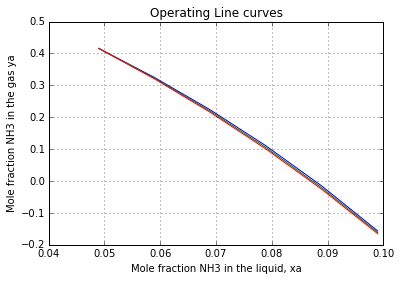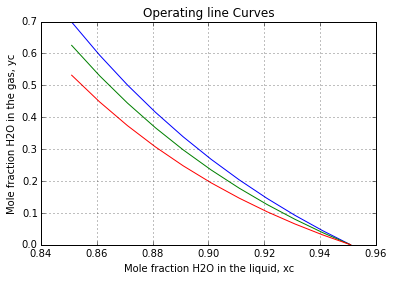The packed depth is:  1.58  m

Ex8.9: Page 327¶

In :
# Illustration 8.9
# Page: 327

print'Illustration 8.9 - Page: 327\n\n'

# solution
from scipy.optimize import fsolve
import numpy
import math
#****Data****#
# C1=CH4 C2=C2H6 C3=n-C3H8 C4=C4H10
Abs=0.15;# [Total absorption,kmol]

T=25;# [OC]
y1=0.7;# [mol fraction]
y2=0.15;# [mol fraction]
y3=0.10;# [mol fraction]
y4=0.05;# [mol fraction]
x1=0.01;# [mol fraction]
x_involatile=0.99;# [mol fraction]
L_by_G=3.5;# [mol liquid/mol entering gas]
#******#

LbyG_top=L_by_G/(1-y2);
LbyG_bottom=(L_by_G+y2)/1;
LbyG_av=(LbyG_top+LbyG_bottom)/2;
# The number of eqb. trays is fixed by C3 absorption:
# For C3 at 25 OC;
m=4.10;
A=LbyG_av/m;
Frabs=0.7;# [Fractional absorption]
X0=0;
# From Eqn. 8.109:
def f43(Np):
return Frabs-((A**Np)-A)/((A**Np)-1)
Np=fsolve(f43,2);
print"Number of trays required is  \n",round(Np,2)
#the answers are slightly different in textbook due to approximation while here answers are precise
Illustration 8.9 - Page: 327

Number of trays required is
3.57